# An Analysis of Spatial Changes Due to Special Relativity

1
1745### Abstract:

This thought experiment addresses loss of volume that occurs when a three-dimensional object is contracted due to relativistic processes. Specifically we investigate the dependence of contraction due to orientation relative to direction of motion. Length contraction states that relative constant velocity will lead to a shortened distance in the direction of motion, when viewed from a separate inertial reference frame. When applied to two dimensional shapes, this results in a reduced area. The problem initially focuses on a square pyramid with the height perpendicular to the direction of motion. Using geometry, it is found that the area of the base is invariant under rotation. The problem is then expanded to general shapes. Any shape can be approximated by inscribing circles within the perimeter, coming arbitrarily close to the shape’s actual area. Since circles are fundamentally invariant under rotation, the change in area of a circle is independent of orientation.  This argument is used to postulate that any two dimensional shape, when contracted in one direction, will have a reduced area that is invariant under rotation. Therefore the contracted area and subsequent reduced volume depend only on the Lorentz factor $$\gamma$$, and not on relative orientation of the object. A key next step is to determine if this can be extrapolated to three dimensions, using spheres instead of circles.

### Introduction:

According to Einstein’s work on special relativity, transformations between inertial reference frames are governed by the following two postulates: First, all laws of physics must remain consistent in all inertial reference frames. Second, the speed of light is constant in all inertial reference frames.

Relativistic processes dictate how systems in our universe behave when traveling close to the speed of light. Length contraction, a relativistic process, is a necessary outcome due to time dilation, in order to preserve the measured relative velocity between two inertial reference frames. The time dilation equation is stated as

\begin{equation}
\Delta{}t^{‘}=\ \gamma{}\Delta{}t
\end{equation}

The contracted length divided by the proper time must yield the same value as the proper length over the dilated time.

\begin{equation}
L=\ \frac{L_o}{\gamma{}}
\end{equation}

with the Lorentz factor, $$\gamma$$, given as

\begin{equation}
\gamma{}=\frac{1}{\sqrt{1-\ {\beta{}}^2}}
\end{equation}

Where $$\Large \beta{}=\ \frac{v}{c}$$

Here L is the length that is perceived from the external reference frame while Lo, the proper length, is the measured length at rest within a given reference frame. This property of length contraction will lead to a reduction in area of two-dimensional figures and a subsequent reduction in volume of three dimensional shapes when measured from an external inertial reference frame.

The question is posed as to whether the orientation of three dimensional objects has an effect on the degree of contraction. Specifically, a square pyramid is considered with the base situated in the plane of motion and the height perpendicular to the direction of motion. See figure 1.

### Geometric Analysis of a Pyramid Under Length Contraction:

The volume of a square pyramid is given as

\begin{equation}
\large
V=\frac{1}{3}A_bH
%eq3
\end{equation}

with Ab the area of the base and H the height.

As stated, dimensions perpendicular to the direction of motion are not contracted, leading to unilateral effects of special relativity. If the pyramid is oriented with one side of the base perpendicular to the direction of motion, $$\theta$$ = 0.0 degrees, the base will contract as shown in figure 1. The resulting contracted base area is found by simply reducing one side by 1/$$\gamma$$.

\begin{equation}\large
A^{‘}=\ w\bullet{}w^{‘}=\ \frac{w^2}{\gamma{}}=\ \frac{A_o}{\gamma{}}
\end{equation}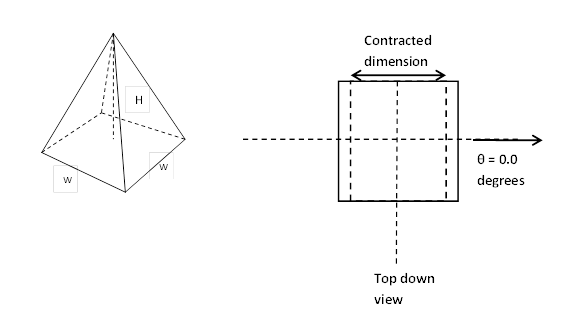Figure 1. A square pyramid with base sides of length w and height H. The top down view shows the contracted dimension of the pyramid base with one side perpendicular to the direction of motion, $$\theta$$ = 0.0 degrees.

Due to symmetry, the meaningful range of orientation is $$\theta$$ = [0o, 45o]. At $$\theta$$ = 45o, the analysis is only slightly more involved. See figure 2. One diagonal dimension is contracted resulting in a parallelogram. The resulting contracted area is found by adding the two resulting equilateral triangles of base $$w\sqrt{2}$$ and height $$\Large \frac{w\sqrt{2}}{2\gamma{}}$$

\begin{equation}\large
A^{‘}=\ w\sqrt{2}\ \frac{w\sqrt{2}}{2\gamma{}}=\ \frac{w^2}{\gamma{}}=\ \frac{A_o}{\gamma{}}
\end{equation}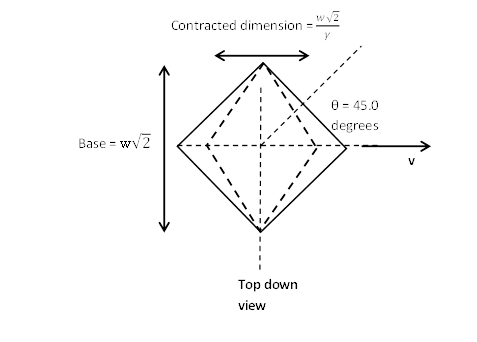Figure 2. Top down view of the pyramid base oriented with the diagonal parallel to the direction of motion, $$\theta$$ = 45 degrees.

When the angle of orientation lies between zero and 45 degrees, the contracted area no longer has equal lengths and therefore involves considerably more math. Equations 6 – 13 give the expressions used to calculate the final contracted area. Again symmetry is used to reduce the problem to matching triangles. See figure 3. Sides L1’ and L2’ are calculated straightforward using length contraction and right angle trigonometry. The remaining side, L3’, is found using the law of cosines. Finally Heron’s formula yields the reduced area. Figure 4 is a plot of the area as a function of orientation using the geometrical approach just described. Included in figure 4 are the lengths of one of the triangle pairs. Note that the solution found here reduces to the limiting cases of zero and forty-five degrees. Also, with $$\gamma$$ = 1, the proper area is regained. The contracted area remains unchanged through the full range of rotation from zero to forty-five degrees.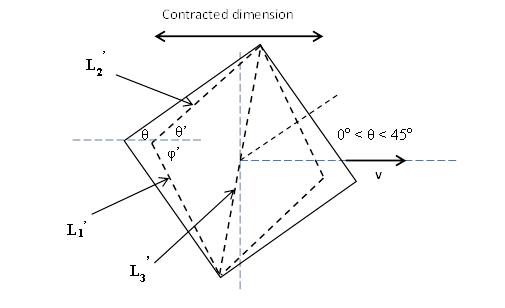Figure 3. Top down view of the pyramid base oriented between zero and 45 degrees to the direction of motion.

\begin{equation} \large
{L_1}^{‘}=w\ \sqrt{\frac{{sin}^2(\theta{})}{{\gamma{}}^2}+\
{cos}^2(\theta{})}\
\end{equation}

\begin{equation} \large
{L_2}^{‘}=w\ \sqrt{\frac{cos^2(\theta{})}{{\gamma{}}^2}+\
{sin}^2(\theta{})}
\end{equation}

\begin{equation}
\large
{\theta ^{\rm{‘}}} = {\sin ^{ – 1}}\left( {\frac{{\gamma \sin \theta }}{{\sqrt {{{\cos }^2}\theta + {\gamma ^2}{{\sin }^2}\theta } }}} \right)
\end{equation}

\begin{equation}
\large
{\varphi ^{\rm{‘}}} = {\sin ^{ – 1}}\left( {\frac{{\gamma \cos \theta }}{{\sqrt {{\gamma ^2}{{\cos }^2}\theta + {{\sin }^2}\theta } }}} \right)
\end{equation}

\begin{equation}
\large
\left( {L_3^{\rm{‘}}} \right) = \frac{w}{\gamma }\sqrt {\left( {1 + {\gamma ^2}} \right) – 2\left( {\cos \theta \sin \theta \left( {1 – {\gamma ^2}} \right)} \right)}
\end{equation}

\begin{equation}
\large
A^{‘}=\frac{1}{2}\sqrt{{{{(L}_1^{‘}}^2+{L_2^{‘}}^2+{L_3^{‘}}^2)}^2+{L_1^{‘}}^4+{L_2^{‘}}^4+{L_3^{‘}}^4}= \frac{{{A_o}}}{\gamma }
\end{equation}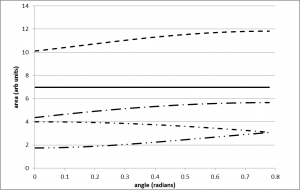Figure 4. Plot of contracted area as a function of base orientation with respect to direction of motion. $$\beta$$ = 0.9/$$\gamma$$ = 2.3. The proper area is 16 (arb units). The plotted lengths represent one triangle and therefore half of the pyramid base.

### Analysis For General Shapes:

It is proposed that any two dimensional closed shape can be comprised of an arbitrarily large number of smaller and smaller inscribed circles. If the number of circles inscribed begins with the largest possible circle, the area of the shape can be described as the sum of the areas of all the circles with i = 1 being the largest. However, ordering the size of the circles is not necessary and in fact may cause loss of precision depending on the shape.

\begin{equation}
\large
A\left( C \right) = \pi \mathop \sum \limits_{i = 1}^\infty  {\left( {{r_i}} \right)^2}
\end{equation}

If the shape is contracted at relativistic speeds, as with the pyramid base, it follows that the circles will become ellipses. See figure 5. The semi-minor axis a represents one half of the contracted dimension of the circle while the semi-major axis b remains the original radius of the circle since it is orthogonal to the direction of motion. Once the shape is contracted, all of the inscribed circles will become ellipses with the new area equation being the sum of all the ellipses.

\begin{equation}
\large
{\left( {\frac{x}{a}} \right)^2} + {\left( {\frac{y}{b}} \right)^2} = 1
\end{equation}

\begin{equation}
\large
A\left(E\right)=\ \pi{}\sum_{i=1}^{\infty{}}r_ia_i
\end{equation}

with ri, the original radius, becoming the semi-major axis.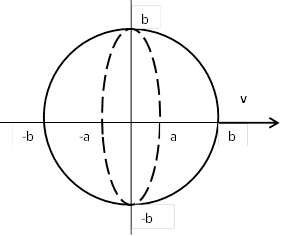Figure 5. A circle before and after contraction due to traveling at relativistic speeds

Then using length contraction for the minor axis, $$\large a_i = r_i/\gamma$$

\begin{equation}
\large
A\left(E\right)=\
\pi{}\sum_{i=1}^{\infty{}}r_i\frac{r_i}{\gamma{}}
\end{equation}

\begin{equation}
\large
A\left(E\right)=\
\frac{\pi{}}{\gamma{}}\sum_{i=1}^{\infty{}}{\left(r_i\right)}^2=A_o/\gamma
\end{equation}

Again, the contracted area reduces to the proper area divided by the Lorentz factor. Due to perfect symmetry of the circle, any rotation of the entire shape, relative to the direction of motion, will only change the direction of contraction while leaving the area of each new ellipse unchanged. It follows that the summation of all of the ellipses will remain invariant under rotation as well.

Figure 6 shows results of a simulation for a square pyramid base at $$\theta$$ = 0.0 degrees as well as rotated 30 degrees relative to the direction of motion. The contracted areas are simulated for both orientations and compared to the known values as well as the proper area. The simulation is run by subtracting the area of an inscribed circle (non-contracted) or an ellipse (contracted) from the original shape. This process reiterates, scaling the new area appropriately. The rotated case takes longer to converge to the correct area since each newly inscribed ellipse takes up less of the remaining region. However after fourteen iterations the simulation data is within 0.10 percent and continues to converge.Figure 6. Simulation results of the area of a square pyramid with base oriented at 30 degrees relative to the direction of motion. $$\beta$$ = 0.40, $$\gamma$$ ~ 1.10. After fourteen iterations, the simulated and actual contracted areas differ by ~ 0.10%.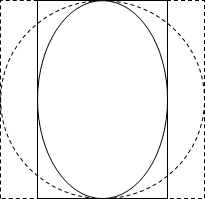Figure 7. The contraction of a circle inscribed inside a square. The original square and circle are dashed lines and the contracted forms are solid lines.

### Discussion:

The contraction will only occur in the direction of motion. It was found that the reduced area of a pyramid’s base will depend on $$\gamma$$ and not the orientation of the base relative to the direction of motion. The problem was expanded to any shape and a more elegant solution is proposed using inscribed circles to estimate the area of any two dimensional closed region. Circles were chosen due to their perfect symmetry and resulting invariance under rotation.

In the future, this problem could be expanded to three dimensions and a similar argument using spheres to replace circles could eliminate the necessity for the shape’s top to be orthogonal to the direction of motion.

### Acknowledgments:

To Mr. Paul McClernon, we owe him our deepest gratitude.  Without his support and guidance over the entire group’s work, we could not have made it this far.  Thank you for helping us no matter how often our dedication seemed to waver.

To Mr. Robert Witz, thank you for helping us with all the ins and outs of the English language.

### Citations:

Einstein, Albert. (1905) “On the Electrodynamics of Moving Bodies.” Translated by John Walker. (1999) Retrieved from http://www.fourmilab.ch/etexts/einstein/specrel/www/

Tipler, Paul Allen, and Ralph A. Llewellyn. Modern Physics. 5th ed. New York, NY: W.H. Freeman, 2008. Print.